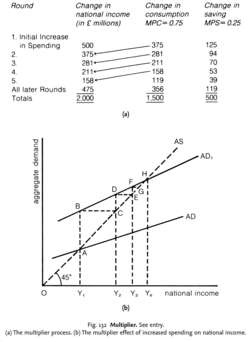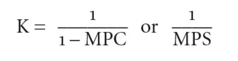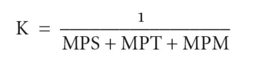# multiplier

(redirected from multiplies)
Also found in: Dictionary, Thesaurus, Medical, Legal, Idioms, Encyclopedia.
Related to multiplies: Multiples

## Multiplier

The investment multiplier which quantifies the overall effects of investment spending on total income. The deposit multiplier which shows the effects of a change in bank deposits on the total amount of outstanding credit and the money supply.

## Multiplier

1. The expression of a currency in terms of one U.S. dollar. For example, if a pound is worth \$2.05 on a given day, it has a multiplier of 2.05. This is also called an American currency quotation. See also: Currency pair

2. In Keynesian economics, the change in income (such as GDP or GNP) that results from a capital injection. For example, if a government spends a certain amount of money building a bridge, it must hire and pay workers to do so. These workers in turn spend their earnings on other goods and services, which fuels economic growth. The multiplier measures how much each dollar the government spends increases economic growth. See also: Demand-side economics.

## multiplierFig. 132 Multiplier. See entry. (a) The multiplier process. (b) The multiplier effect of increased spending on national income.

## multiplier

the ratio of an induced change in the EQUILIBRIUM LEVEL OF NATIONAL INCOME to an initial change in the level of spending. The ‘multiplier effect’ denotes the phenomenon whereby some initial increase (or decrease) in the rate of spending will bring about a more than proportionate increase (or decrease) in national income. Two important features of the multiplier need to be noted:
1. it is a cumulative process rather than instantaneous in effect and, as such, is best viewed in terms of a series of successive ‘rounds’ of additions to income;
2. the value of the multiplier depends on the fraction of extra INCOME that is spent on CONSUMPTION (the MARGINAL PROPENSITY TO CONSUME) (MPC) at each successive round.

For simplicity, let us assume that all income is either consumed or withdrawn as SAVINGS. (That is, the MPC and MARGINAL PROPENSITY TO SAVE (MPS) together = 1.) The value of the multiplier (K) is then given by the formula:The larger an increase in consumption from an increment of income, the larger the multiplier. Thus, if MPC is 0.9 and MPS is 0.1, the multiplier value is 10; if MPC is 0.75 and MPS is 0.25, the multiplier value is only 4.

The multiplier effect is illustrated in Fig. 132 (a) and. With a multiplier value of 4, an initial £500 million of extra spending results in a £2 billion increase in national income, as Fig. 132 (a) shows. In each round, a proportion of the additional income created is saved and so leaks from the circular flow, failing to get passed on as additional consumption expenditure in the next round. When the cumulative total of these savings leakages is equal to the initial increase in spending, the multiplier process ceases and the economy reaches a new equilibrium.

Fig. 132 (b) demonstrates the multiplier effect in graphical form. Starting at national income level OY1, if AGGREGATE DEMAND increases from AD to AD1, then the initial injection of extra spending AB would serve to increase output and income by Y1Y2. This additional income would induce yet more spending (CD), which would in turn increase output and income by Y2 Y3. This additional income would induce yet more spending (EF), which would in turn increase output and income yet further, and so on. The process ends when the new equilibrium level of income Ye is reached.

In addition, of course, to the savings ‘withdrawal’ from the income flow, there are also TAXATION and IMPORT withdrawals that further reduce the value of the multiplier. Thus, the more sophisticated multiplier is given by the formula:where MPT is the MARGINAL PROPENSITY TO TAX and MPM is the MARGINAL PROPENSITY TO IMPORT. See also CIRCULAR FLOW OF NATIONAL INCOME MODEL, ACCELERATOR.

Collins Dictionary of Economics, 4th ed. © C. Pass, B. Lowes, L. Davies 2005
Site: Follow: Share:
Open / Close